Welcome back to our Examination Paper Analysis series!

In this article, we will be reviewing the 2018 Xinmin Primary School (XMPS) P5 SA2 Science Examination Paper.

## What Topics Are Covered In This Paper?As seen from the topics listed above, this paper includes questions from quite an even spread of lower and upper block topics, unlike other school papers that test more questions from the lower block topics instead.

Another interesting aspect of this paper is that the main topic tested is Energy, which is commonly taught in P6 in various schools. Out of the 28 multiple-choice questions in Booklet A, there are 9 questions on this topic. In Booklet B, almost 25% of the open-ended questions are also on this topic.

✳️ Please note that the topic of Energy is about explaining energy conversions and is actually different from the topic of Energy in Food which is about the process of photosynthesis.

As I typically cover P4 & P5 topics in our other examination paper analysis articles, I will be discussing 2 questions on the topic of Energy instead.

## Let’s Take A Look At Q28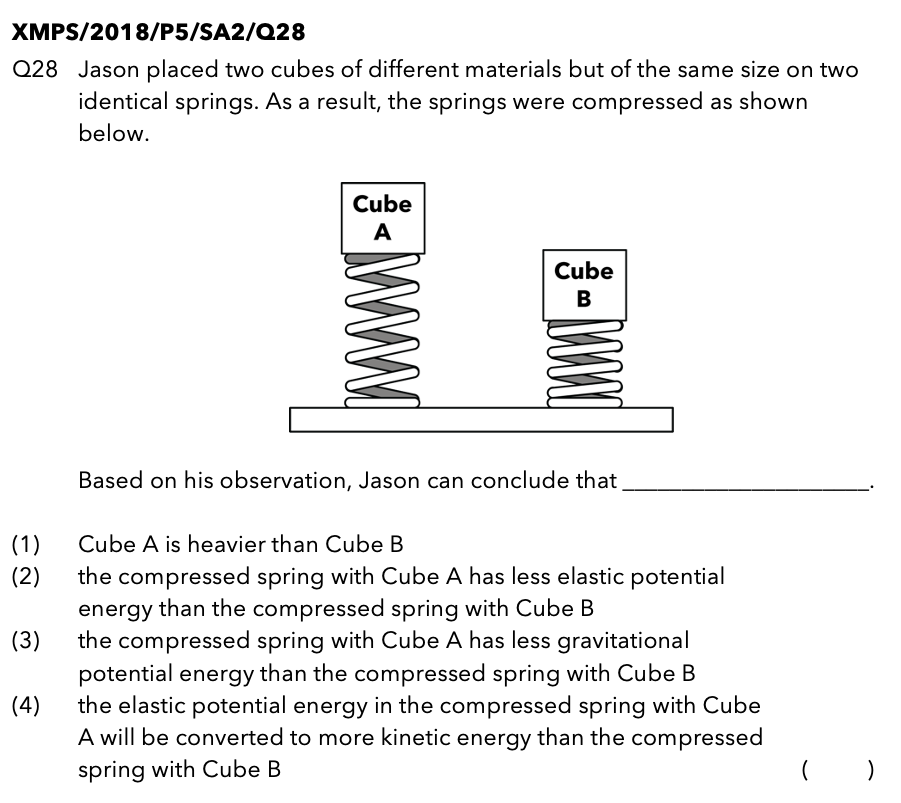Source: Xinmin Primary School – 2018 P5 SA2 Examination Paper [Q28]

In this multiple-choice question, it is mentioned that “Jason placed two cubes of different materials but of the same size on two identical springs. As a result, the springs were compressed as shown below.”

What are the important phrases in this sentence to take note of?

• “two cubes of different materials but of the same size”
Think of a styrofoam block and a metal block of the same size – would they have the same mass? No, the metal block will be much heavier than the styrofoam block! Thus, this phrase suggests that the cubes are of different mass.
• “identical springs”
This phrase indicates that the springs are of the same mass and stiffness.

## Thought ProcessAs seen from the diagram above, the spring with Cube A on it (Spring A) is longer than the spring with Cube B on it (Spring B) after the cubes were placed on them. This suggests that Spring A is less compressed than Spring B. Based on this observation, what can you conclude about the mass of Cube A? We will be able to conclude that Cube A is lighter than Cube B.

Imagine a baby and a sumo wrestler sitting on a bed. Who would cause the bed to depress more? The answer is the sumo wrestler! The sumo wrestler is much heavier than the baby which would cause the bed to depress more as compared to the baby.

Let’s discuss the type of energy that the springs and cubes in this question possess when the springs are stretched or compressed.

Since both springs are compressed, they possess elastic potential energy (EPE). Are you able to recall the 2 factors that affect the amount of EPE a spring possesses?

1. The extent to which (how much) the spring is stretched or compressed.
2. The stiffness of the spring.

The question states that both springs are identical which means that both springs have the same stiffness. Thus, since Spring A is compressed less than Spring B, Spring A possesses less EPE as compared to Spring B.

Food for Thought: Do the cubes possess EPE?

If your answer is no, you are absolutely correct! The cubes do not possess EPE as they are not stretched or compressed in any way.

What is another type of energy that the springs and cubes possess? Are you thinking of gravitational potential energy (GPE)? Let’s recall the 2 factors that affect the amount of GPE together!

1. Mass of the object
The greater the mass of the object, the greater the amount of gravitational potential energy possessed by the object.
2. Height of the object from the ground
The greater the height of the object from the ground, the greater the amount of gravitational potential energy possessed by the object.

Let’s take a look at the cubes first. In order for an object to possess GPE, the object must be at a height above the ground. Since both cubes are at a height above the ground, both cubes possess GPE.

Based on the diagram given, are we able to compare the amount of GPE possessed by each cube?The answer is no! As we’ve determined earlier, Cube A is lighter (Spring A is compressed less), however, it is at a greater height above the ground. On the other hand, Cube B is heavier (Spring B is compressed more) but is at a lower height above the ground. Since there are 2 changed variables, we will not be able to compare the amount of GPE possessed by both cubes.

What about the amount of GPE possessed by both springs? Many students may respond with:

“From the diagram, we can see that Spring A is compressed less, which means that Spring A is longer (taller) than Spring B and would possess more GPE.”

This is actually a misconception! When we are considering the “height of the object above the ground”, we are measuring the gap between the bottom of the object to the ground and NOT measuring the height of the object itself.

Here’s another diagram to explain this: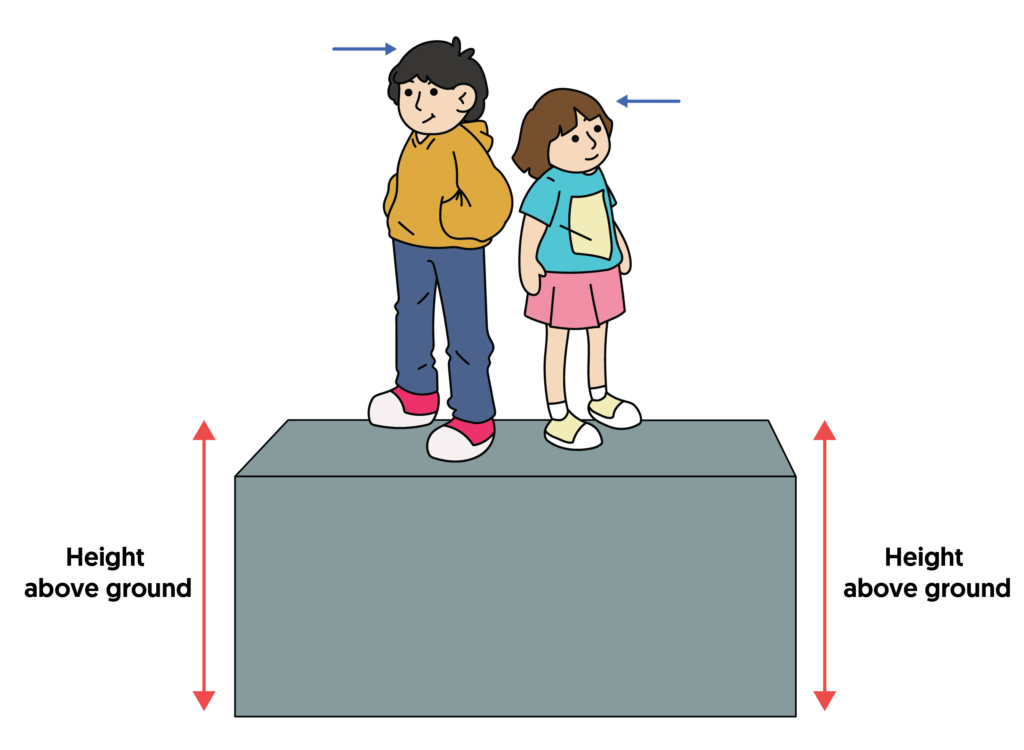Both the boy and the girl are standing on a stage. Although the boy is taller than the girl (as indicated by the blue arrows), they are both still standing on the stage. Thus, both the boy and the girl are at the same height above the ground (as indicated by the red arrows).With reference to this example, although Spring A is longer (taller) than Spring B, both springs are still at the same height above the ground (as illustrated by the red arrows in the diagram below) and possess the same amount of gravitational potential energy.

Let’s take a look at the options to answer the question: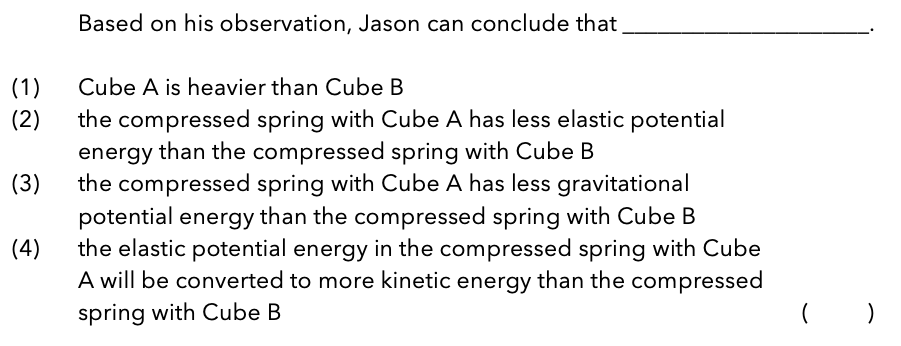### Option 1: Cube A is heavier than Cube B

From the diagram given, we can see that Spring A is less compressed than Spring B, which suggests that Cube A is lighter than Cube B.

Thus, Option (1) is incorrect.

### Option 2: the compressed spring with Cube A has less elastic potential energy than the compressed spring with Cube B

As mentioned earlier, EPE possessed by the spring depends on:

1. The extent to which (how much) the spring is stretched or compressed.
2. The stiffness of the spring.

Since the question mentions that both springs are identical, this means that both springs have the same stiffness. As Spring A is compressed less, Spring A possesses less EPE than Spring B.

Thus, Option (2) is correct.

Let’s review the remaining options to be sure of our answer and understand why they are incorrect.

### Option 3: the compressed spring with Cube A has less gravitational potential energy than the compressed spring with Cube B

This option is commonly chosen by students who have the misconception that a longer (taller) spring will possess more GPE because it is at a greater height above the ground. As explained earlier, both springs actually possess the same amount of GPE as both springs are the same height above the ground.

Thus, Option (3) is incorrect.

### Option 4: the elastic potential energy in the compressed spring with Cube A will be converted to more kinetic energy than the compressed spring with Cube B

As Spring A is compressed less than Spring B and possesses less EPE, this means that less EPE of Spring A will be converted to less kinetic energy (KE) of Cube A when Spring A is released as compared to Cube B.

Thus, Option (4) is incorrect.

The correct answer to this question is Option (2).

## Let’s Take A Look At Q40b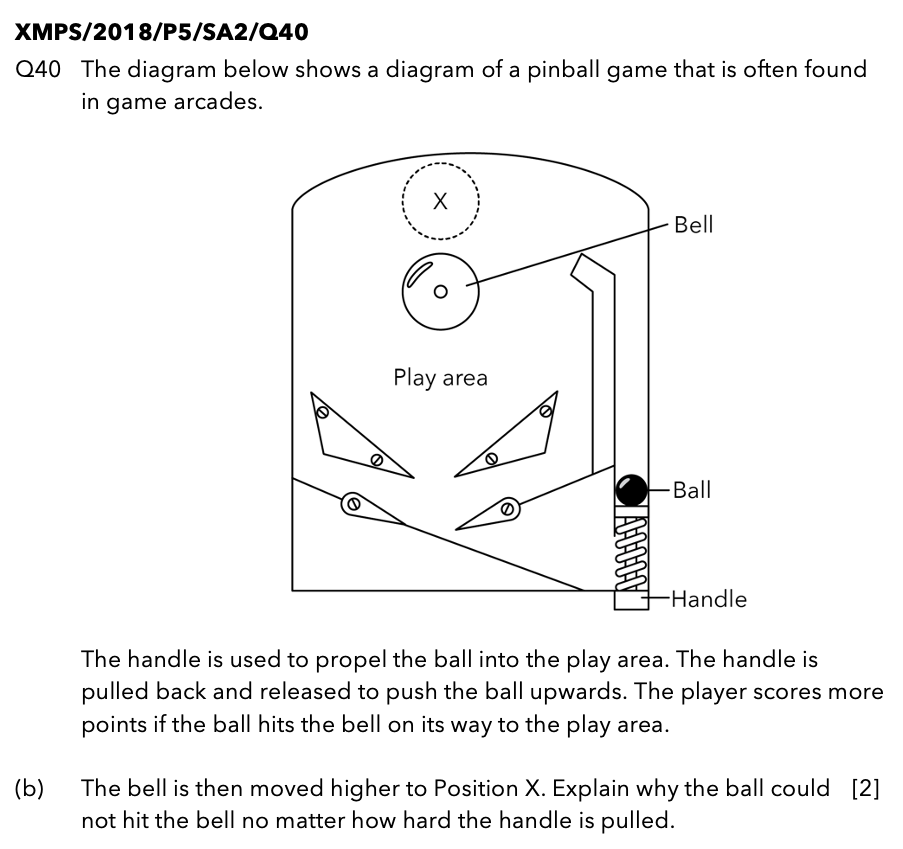Source: Xinmin Primary School – 2018 P5 SA2 Examination Paper [Q40b]

This question is about a pinball game. You may have played virtual pinball computer games before, but do you know how the actual pinball game works in real life?

The aim of the pinball game is to propel the ball into the play area and obtain as many points as possible by letting the ball hit the obstacles in the play area and at the same time, preventing the ball from falling between the flippers and out of the play area.

In order to propel the ball into the play area, the handle is pulled back and released. As seen in the diagram, pulling the handle downwards will cause the spring attached to it to be compressed, which will help to propel the ball upwards into the play area.

## Thought Process

Now that we understand how the game works, let’s discuss the energy conversions involved in the pinball game.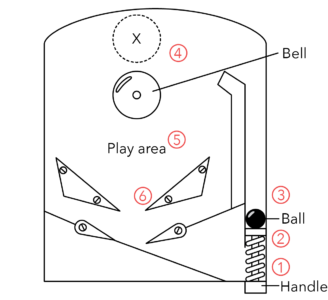I’ve labelled the diagram with Points 1 to 6 in order for us to identify the energy conversions that take place during the different parts of the game.

### Point 1

When the handle is pulled back, the spring will be compressed and will possess elastic potential energy (EPE).

### Point 2

When the handle is released, the EPE of the spring will be converted to kinetic energy (KE), allowing the spring to return to its original shape.

### Point 3

As the KE of the spring is transferred to the KE. of the ball, the ball will be propelled upwards into the play area.

### Point 4

Since the ball is moving upwards, the KE of the ball will be converted to gravitational potential energy (GPE).

### Point 5

As the ball continues to move downwards in the play area, the GPE of the ball will be converted to KE.

### Point 6

As the ball hits the obstacles in the play area, the KE of the ball will be converted to heat energy (HE) and sound energy (SE) due to friction between the surfaces of the ball and the obstacles in the play area.

By combining these together, we have worked out the energy conversions involved in the pinball game.Let’s take a look at the question again: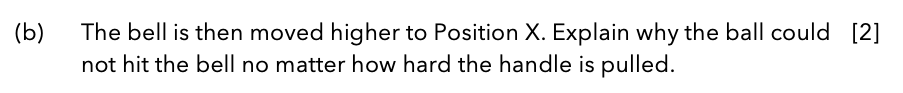In order for the ball to hit the bell at Position X, which is higher, the ball will need to travel to a greater height. Are you able to recall the type of energy that is affected by the height of the object above the ground? If your answer is GPE, you are correct!

For the ball to travel to a greater height, the ball will need to possess more GPE.

By using the energy conversion diagram, the spring will need to possess more EPE from the start (Point 1) in order for the ball to possess more GPE (Point 4) to reach a greater height.As mentioned earlier in this article, here are the 2 factors that affect the amount of EPE in the spring:

1. The extent to which (how much) the spring is stretched or compressed.
2. The stiffness of the spring.

In order for the spring to possess more EPE, the spring will either need to be compressed more OR be less stiff. Since the spring was not changed in the pinball machine, the stiffness of the spring will remain the same. Thus, in order for the spring to possess more EPE, the spring will need to be compressed more.

We will need to pull the handle harder in order to compress the spring more. However, as you may have realised, no matter how hard the handle is pulled, there is a limit to how much the spring can be compressed. Thus, there will be a limit to the amount of EPE the spring can possess.

When the handle was pulled to compress the spring fully, the spring was still not able to possess enough elastic energy. Thus, the amount of elastic potential energy that was converted into kinetic energy of the spring was insufficient before being transferred to the ball. Finally, the ball did not possess enough kinetic energy to be converted to gravitational potential energy, preventing the ball from reaching the bell at Position X.

## In Conclusion

1. Identify the important keywords/keyphrases in the question.
2. Understand that the size of the object does not always relate to its mass.
3. Understand that “the height of the object” is different from “the height of the object from the ground”.
4. Explain how the pinball game works in real life using energy conversion.

We have come to the end of the examination paper analysis of 2018 Xinmin Primary School (XMPS) P5 SA2 Science Examination Paper.

Stay tuned for more exam paper analysis coming your way! 😊